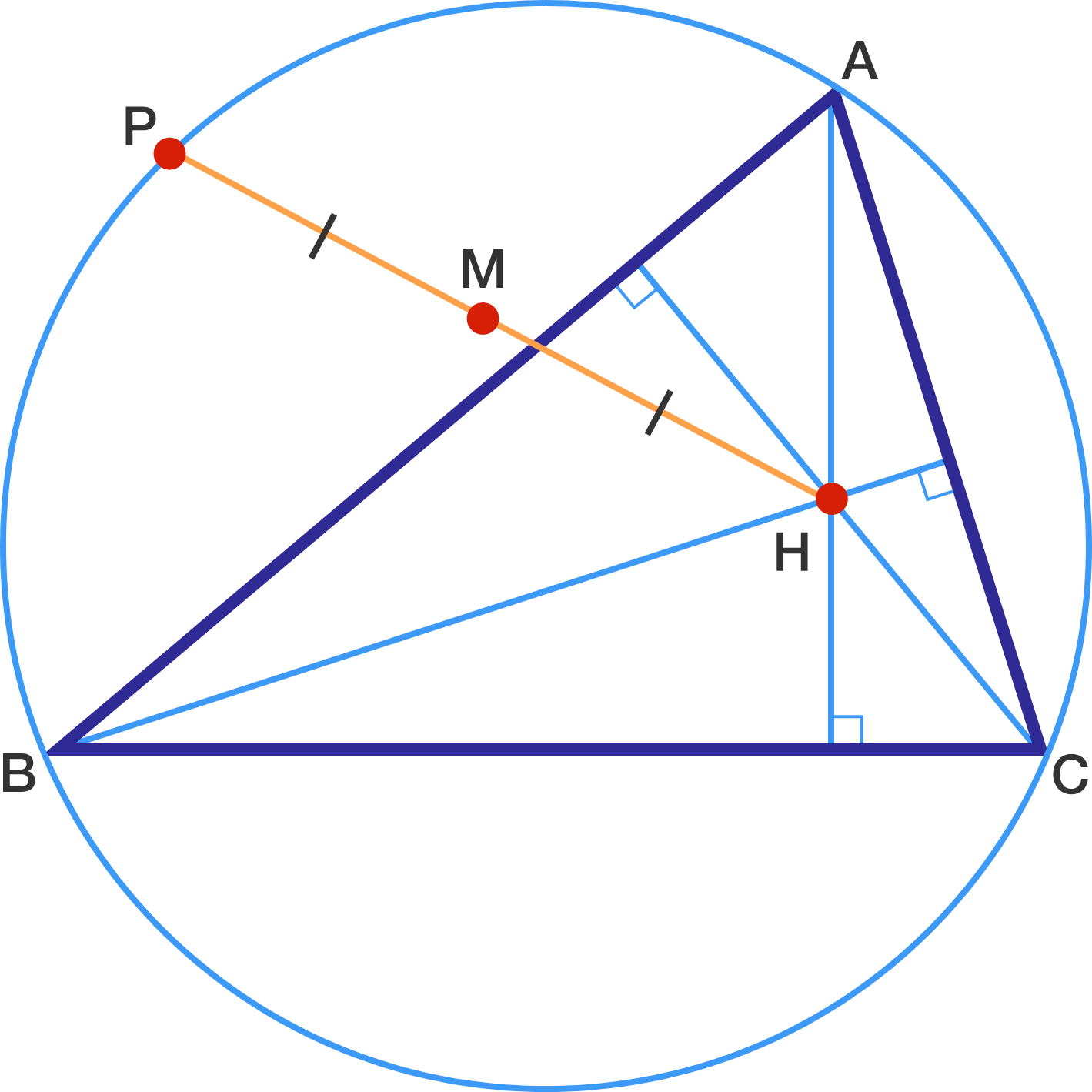# Drawing Circles Isn't Enough!

Geometry Level 5

Let $H$ be the orthocenter of triangle $ABC$, which has a circumradius of 4.
Let $P$ be a point on the circumcircle of triangle $ABC$.
Let $M$ be the midpoint of $HP$.

What is the area of the locus enclosed by $M$ as $P$ moves along the circumcircle?

Give your answer to 2 decimal places.Bonus: What specific shape is this locus also known as?

If you think there is no set answer, type 13.37 as your answer.

×

Problem Loading...

Note Loading...

Set Loading...